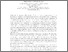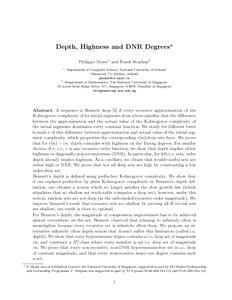# Depth, Highness and DNR Degrees

Moser, Philippe and Stephan, Frank (2015) Depth, Highness and DNR Degrees. In: FCT 2015 : 20th International Symposium on Fundamentals of Computation Theory, 17-19 August 2015, Gdansk, Poland.Previewmore...Add this article to your Mendeley library

## Abstract

A sequence is Bennett deep  if every recursive approximation of the Kolmogorov complexity of its initial segments from above satisfies that the difference between the approximation and the actual value of the Kolmogorov complexity of the initial segments dominates every constant function. We study for different lower bounds r of this difference between approximation and actual value of the initial segment complexity, which properties the corresponding r(n)-deep sets have. We prove that for r(n) = εn, depth coincides with highness on the Turing degrees. For smaller choices of r, i.e., r is any recursive order function, we show that depth implies either highness or diagonally-non-recursiveness (DNR). In particular, for left-r.e. sets, order depth already implies highness. As a corollary, we obtain that weakly-useful sets are either high or DNR. We prove that not all deep sets are high by constructing a low order-deep set. Bennett's depth is defined using prefix-free Kolmogorov complexity. We show that if one replaces prefix-free by plain Kolmogorov complexity in Bennett's depth definition, one obtains a notion which no longer satisfies the slow growth law (which stipulates that no shallow set truth-table computes a deep set); however, under this notion, random sets are not deep (at the unbounded recursive order magnitude). We improve Bennett's result that recursive sets are shallow by proving all K-trivial sets are shallow; our result is close to optimal. For Bennett's depth, the magnitude of compression improvement has to be achieved almost everywhere on the set. Bennett observed that relaxing to infinitely often is meaningless because every recursive set is infinitely often deep. We propose an alternative infinitely often depth notion that doesn't suffer this limitation (called i.o. depth).We show that every hyperimmune degree contains a i.o. deep set of magnitude εn, and construct a π01- class where every member is an i.o. deep set of magnitude εn. We prove that every non-recursive, non-DNR hyperimmune-free set is i.o. deep of constant magnitude, and that every nonrecursive many-one degree contains such a set.

Item Type: Conference or Workshop Item (Paper) Depth; Highness; DNR Degrees; Faculty of Science and Engineering > Computer Science 6516 Philippe Moser 03 Nov 2015 16:59 YesItem control page